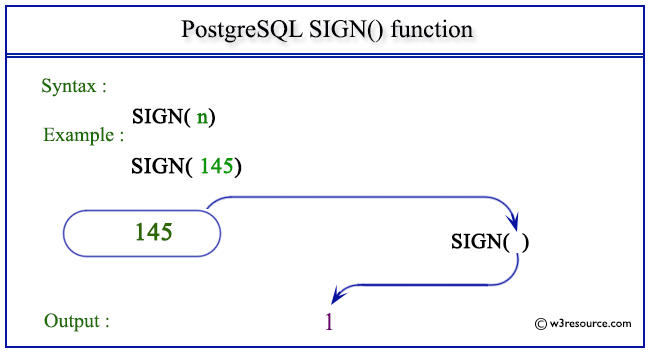# PostgreSQL SIGN() function

## SIGN() function

The PostgreSQL sign() function is used to return the sign of a given number. It returns 1 if the number is positive and -1 if negative.

Syntax:

```sign()
```

PostgreSQL Version: 9.3

Pictorial presentation of PostgreSQL SIGN() functionExample: PostgreSQL SIGN() function

Code:

``````SELECT SIGN(14.321) AS "Positive Number";
```
```

Sample Output:

``` Positive Number
-----------------
1
(1 row)
```

Example : PostgreSQL SIGN() function using negative value

Code:

``````SELECT SIGN(-14.321) AS "Negative Number";
```
```

Sample Output:

``` Negative Number
-----------------
-1
(1 row)
```

Previous: ROUND function
Next: SIN function

﻿